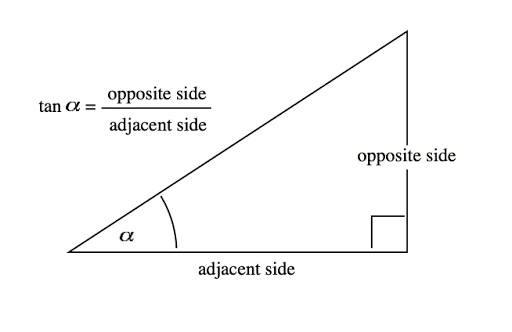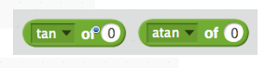## Wednesday, December 23, 2020

### Addendum to Scalene Triangle Mouse I

At the conclusion of the Coding the Scalene Triangle Mouse I document, I described what I considered to be a flaw in the code; when completing the third leg of the triangle the mouse did not actually point to the starting point of the triangle but moved in a skewed fashion.
Scratch does contain a [point towards (mouse-pointer or another sprite)] block.

I suppose one could make the starting point of the triangle a small circular sprite and then simply ‘point to it’ to draw the third leg of the triangle. I call this approach a work-around as it solves the problem for the present but when time allows a more elegant solution will be found. So I thought about how to create code that will point the mouse from one point to any other specified point. In other words, I wanted to code a generalized solution. It turns out that the basic trigonometry operator, tangent (angle) abbreviated tan(angle) helps to solve the problem.In Scratch, the tan operator (see below) returns the numerical ratio of the opposite side over adjacent side as shown in the diagram above.
The arctan operator takes the numerical ratio and returns the angle.
For example, for a 38º angle, tan 38º = 0.7812 and the arctan of 0.7812 = 38ºIn the diagram below the xpos and ypos reporters in Scratch return the values for x2 and y2. The values for x1 and y1 are set in the code. These values are used to compute the tangent of the angle and then the arctangent operator computes the value in degrees of the angle. The mouse can then be turned to the left through the angle (90º – angle)  to be pointed directly towards the point (x1, y1).

I have written a detailed tutorial describing the coding of the above addendum to the Scalene Mouse Triangle project. A free PDF file of this document that might be useful to both teachers and students can be obtained by emailing me at grandadscience@gmail.com.

## Monday, December 21, 2020

### Pen Commands in Scratch 3

Go to http://scratch.mit.edu/ and click on Create in the upper left corner. The Editor page, shown below, will open. This version is Scratch 3. Earlier versions of Scratch had a Pen tab listed with the other colored categories of blocks. Scratch 3 has moved the Pen commands.
To find them, note the small blue block in the lower left of the Scratch home screen. The blue icon indicates additional blocks. Click on the blue icon and a page of additonal commands are revealed.

Click on the Pen icon in the lower left corner of the above menu to reveal a page of options. Choose the option Pen.
If you use Pen commands in a project, every time you open the project the Pen icon will appear under the My Blocks menu icon.

A copy of this post in PDF file format suitable for teacher or student use can be obtained, free of charge, by sending an email request to grandadscience@gmail.com.

### Coding Math Concepts as Tests of Student Comprehension

In Bloom's Taxonomy of Educational Objectives, a test of a learner's 'comprehension' of a concept is the ability of the learner to 'rephrase’ or ‘restate' the concept in as many different ways as possible. For several years I have been interested in using standard mathematics curriculum as a source of computer coding exercises in which students ‘rephrase’ math concepts. Geometry is an especially rich source for computer coding projects. In this post we use computer code to explore the scalene triangle concept.

Where to begin?

Scalene triangle: A triangle with three unequal side lengths and three unequal angle measures

The definition tells us how to recognize a scalene triangle if we know the lengths of the three sides.  But the definition is static, not dynamic. Typing the definition into computer code will code the individual letters (ASCI II) but the code simply displays the definition as text, not as a figure of a scalene triangle.

This is often a point of frustration with beginning programmers as they may know a ‘concept’ as a set of words or symbols but become frustrated when trying to code the concept.

To code a scalene triangle in Scratch is not a trivial exercise.

To begin, I’ve created a starter project that has a mouse as sprite 1. You can click on this link to download the project or create your own starter.

Scalene Triangle Mouse Starter

https://scratch.mit.edu/projects/170195620/

Scalene Triangle Mouse I

https://scratch.mit.edu/projects/463821115/

I am in the process of writing a detailed tutorial describing the coding of the Scalene Triangle Mouse I project. A free PDF file of this document that might be useful to both teachers and students can be obtained by emailing me at grandadscience@gmail.com

The following projects are in the same spirit as each project codes a math concept.

https://scratch.mit.edu/projects/465028285/

Perpendicular Mouse

https://scratch.mit.edu/projects/20186064/

Parallel Mouse

https://scratch.mit.edu/projects/21653980/

Angle Mouse

https://scratch.mit.edu/projects/16793255/